# Early Attempts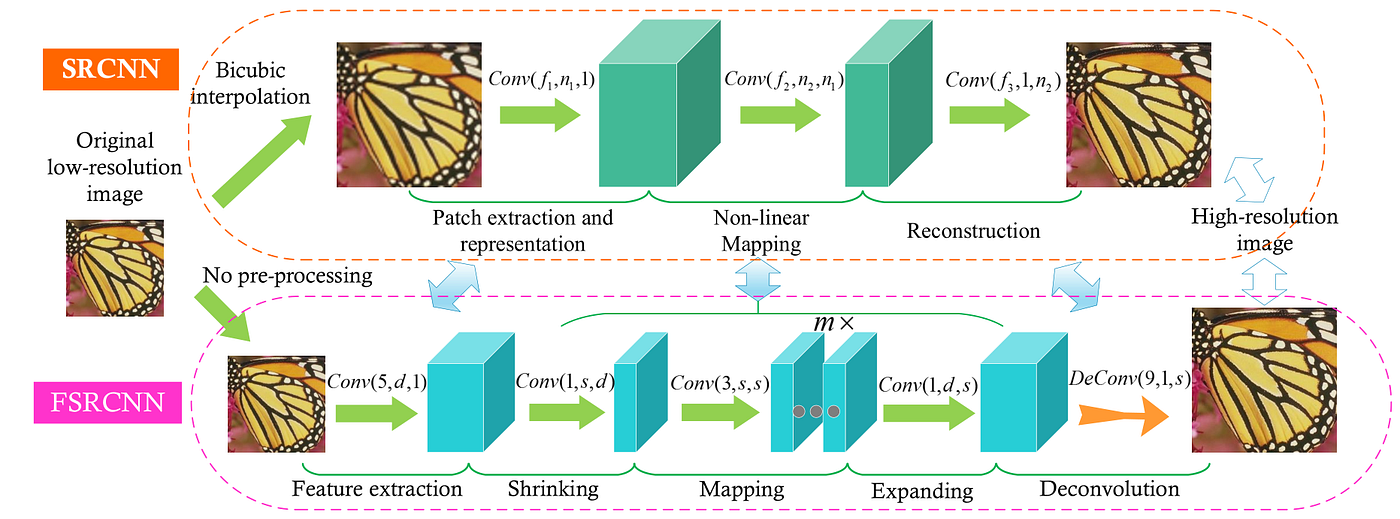Figure 1. The network structures of SRCNN and FSRCNN. The SRCNN network aims to learn an end-to-end mapping from an upsampled version of the input LR image (using the bicubic algorithm) to the HR target. Instead, FSRCNN introduces a deconvolution layer as the last layer of the network while replacing the non-linear mapping section with a three-step process: shrinking, mapping, and expanding. The authors refer to this as an “hour-glass” structure.

# Methods

## Residual Dense Network (RDN)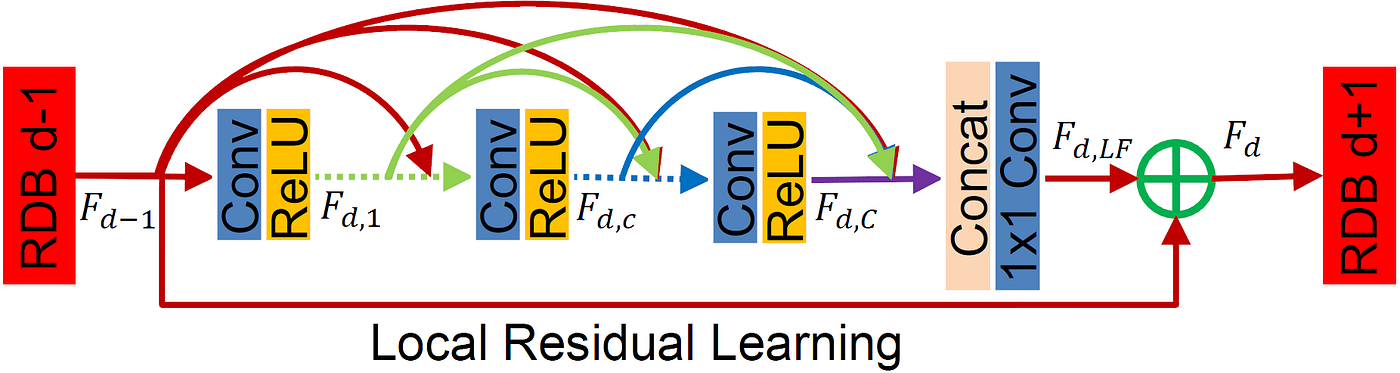Figure 2. Residual Dense Blocks, the main building block of RDN.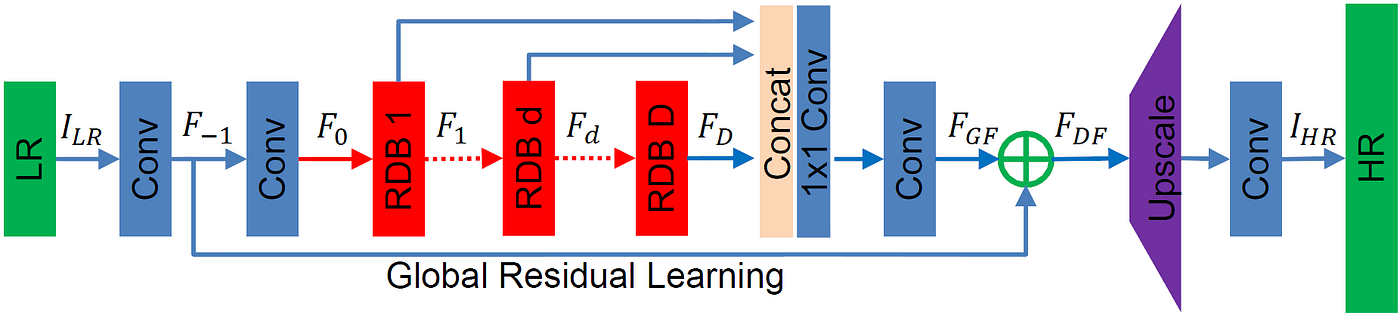Figure 3. The architecture of RDN.

## Residual Channel Attention Network (RCAN)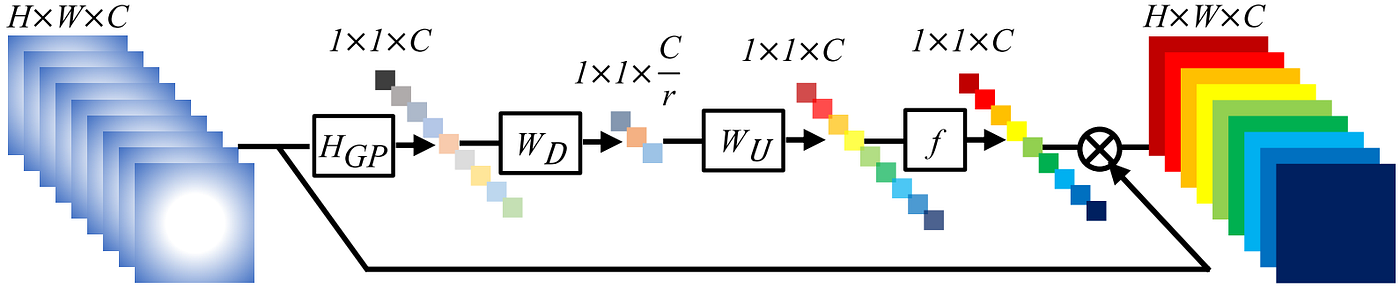Figure 4. Channel Attention mechanism introduced in .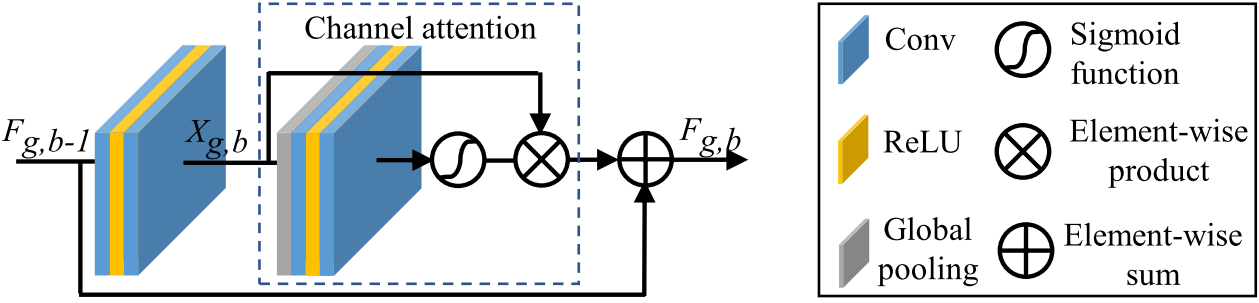Figure 5. The structure of RCABs.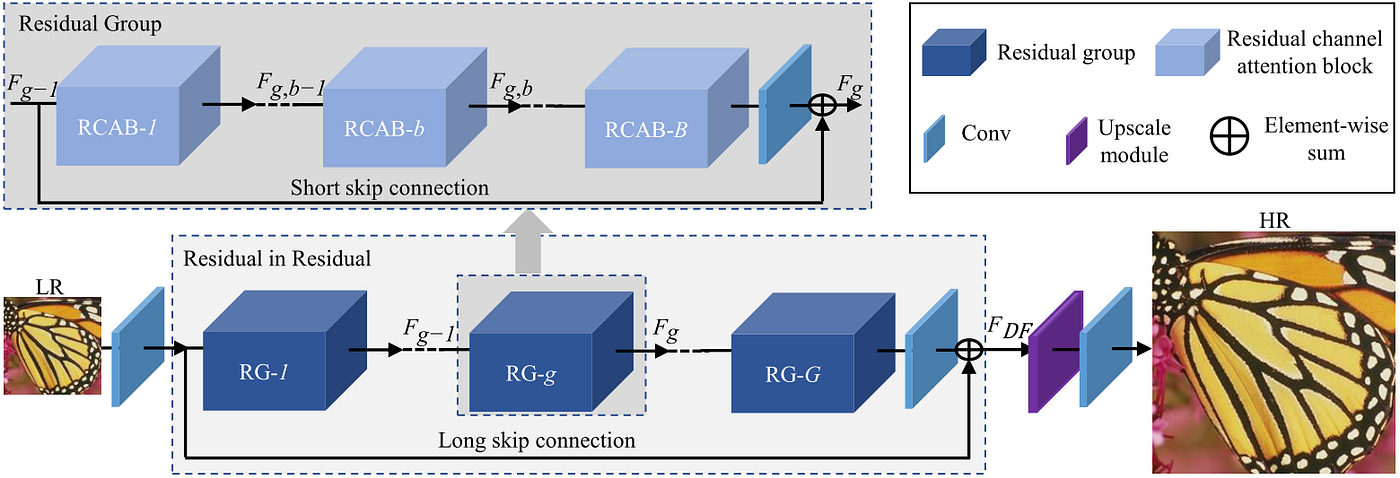Figure 6. The architecture of RCAN.

## Super-Resolution Feedback Network (SRFBN)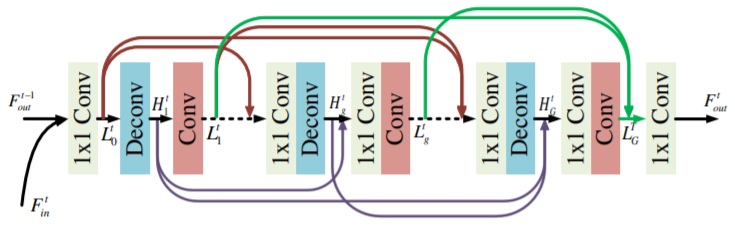Figure 7. The structure of FB.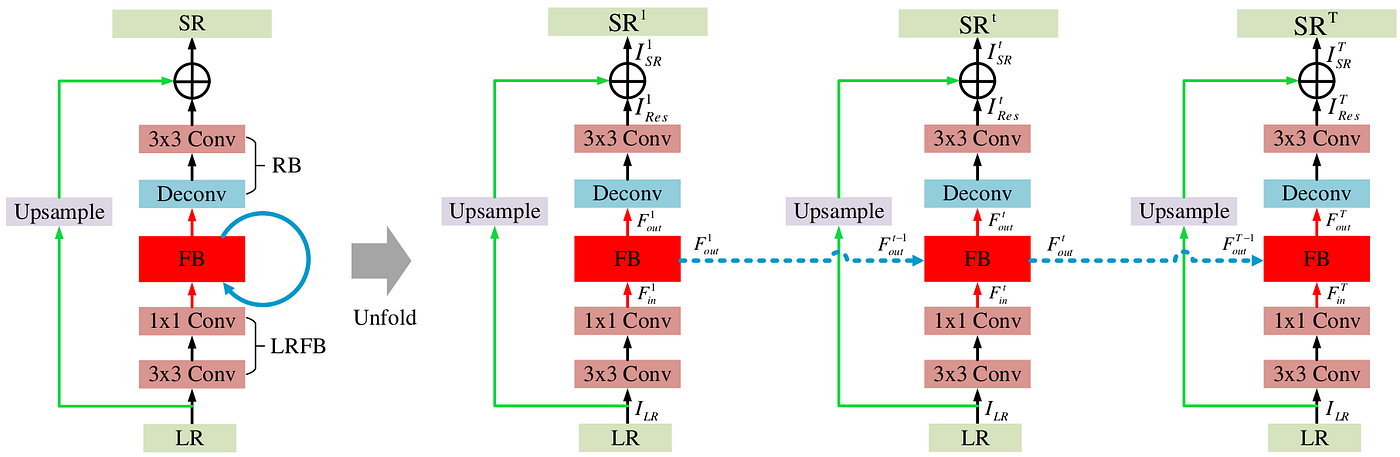Figure 8. The structure of SRFBN (to the left) and unfolded (to the right). Layers labeled as LRFB are the LR Feature extraction Block, and the couple labeled as RB is the Reconstruction Block, which maps the extracted features into an RGB image.

## Densely Residual Laplacian Network (DRLN)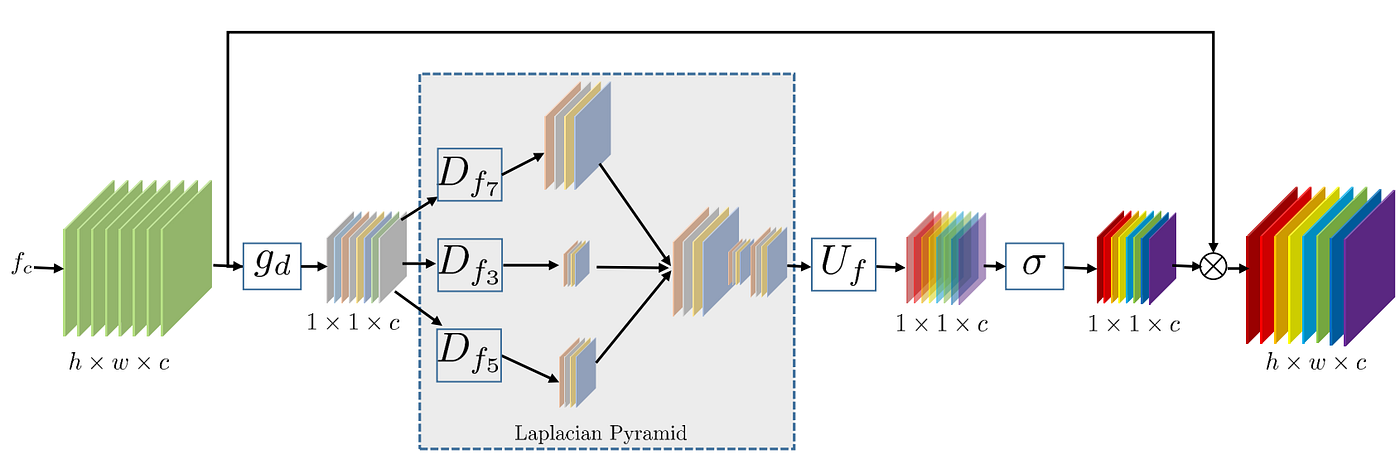Figure 9. The structure of the Laplacian Attention mechanism. The subscripts in the Laplacian Pyramid denote the dilation factor of the respective convolution layers.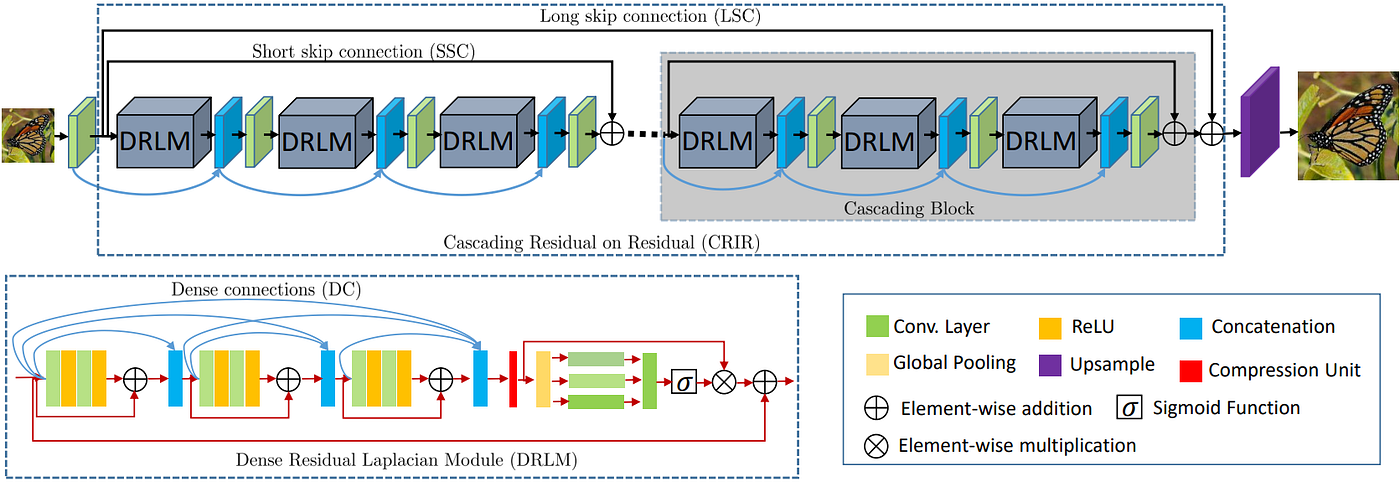Figure 10. The structure of DRLN. In this figure, residual connections are referred to as skip connections.

## Gated Multiple Feedback Network (GMFN)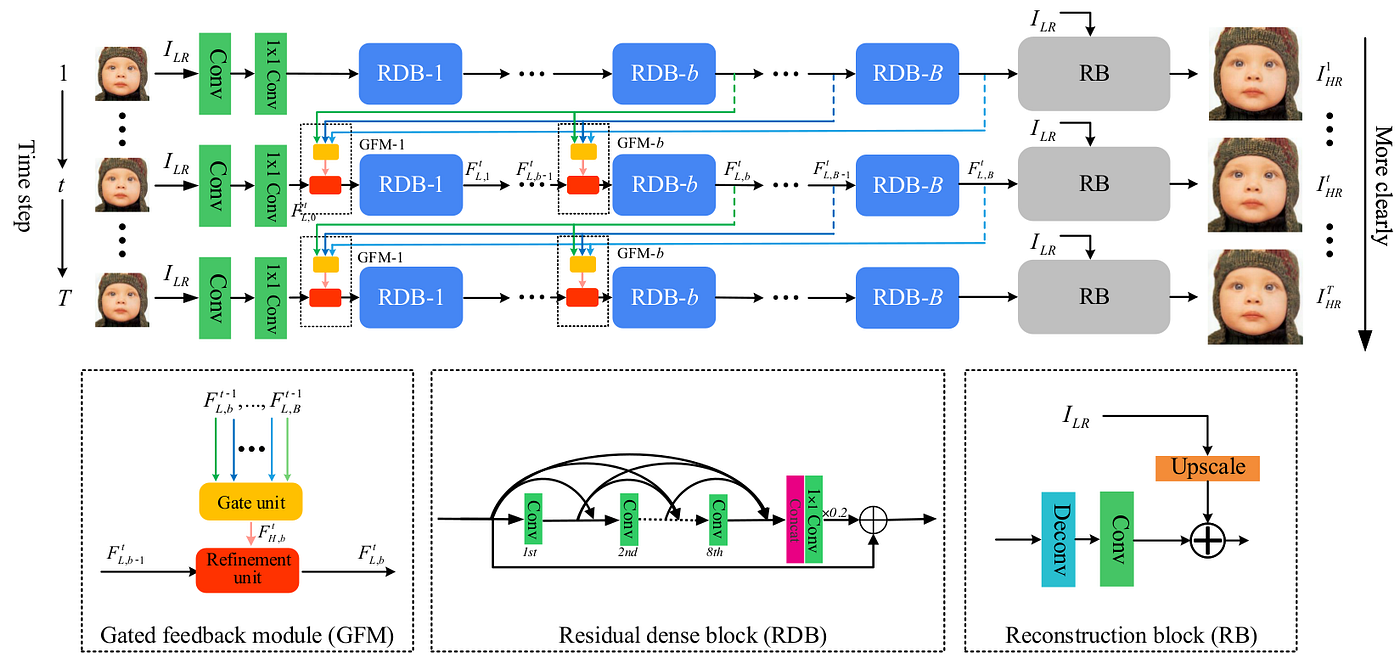Figure 11. The structure of GMFN, GFM, RDB (identical to the one introduced in RDNs), and the reconstruction block (identical to the final layers in the SRFBN model).

## Cross-Scale Non-Local Network (CSNLN)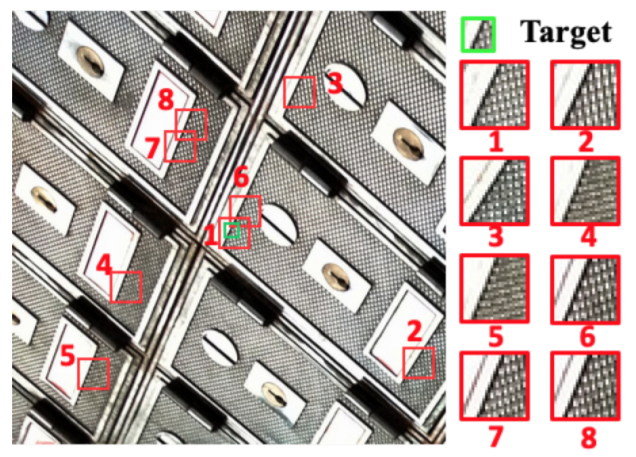Figure 11. Non-local cross-scale similarities in an image.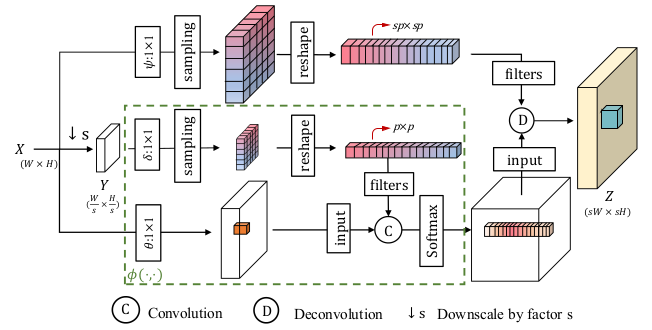Figure 12. The structure of the CSNLA mechanism. The upper branch looks for the patches in the LR image corresponding to patches in the HR image, while the branches within the green box find the similarities.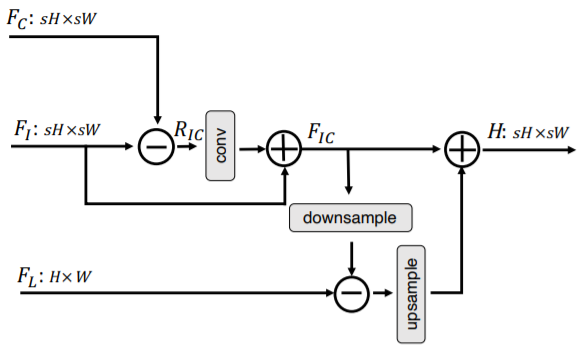Figure 13. The mutual-projected fusion. The upsample operation, as well as the downsample operation, are implemented using convolutional layers. The lower input denoted as L is the features extracted by the convolution layers processed by the attention mechanisms. s refers to the scale factor for the SR transformation.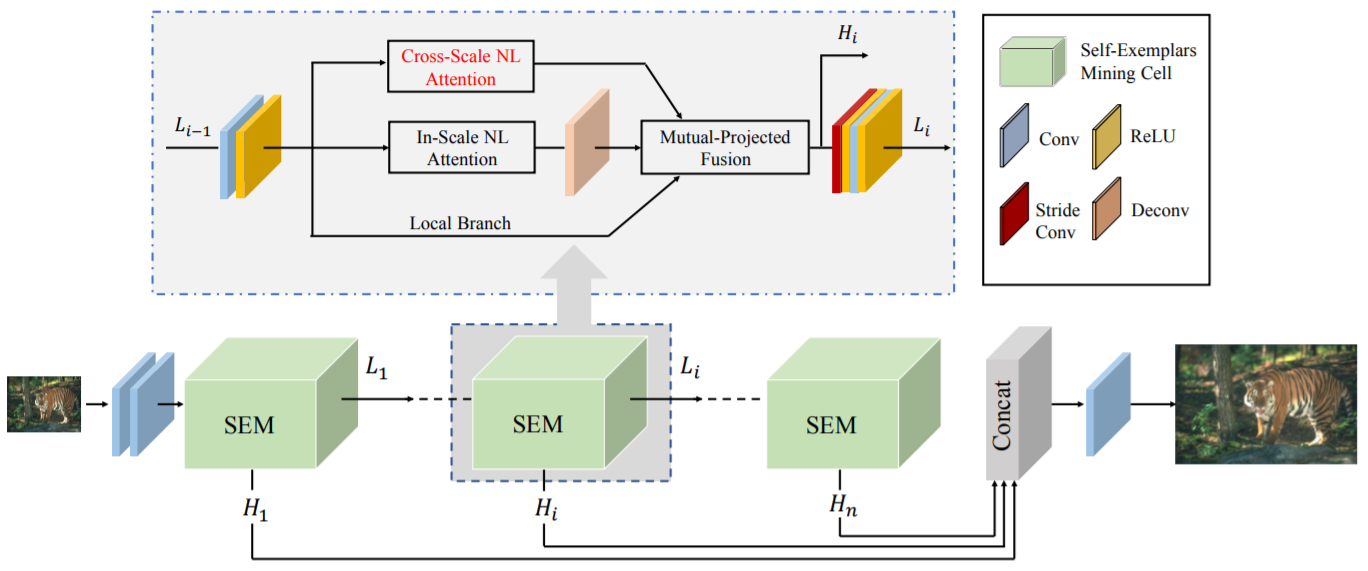Figure 14. The unfolded structure of CSNLN. Features extracted with each iteration are collected and concatenated into the same tensor.

# Performance Evaluation and Comparison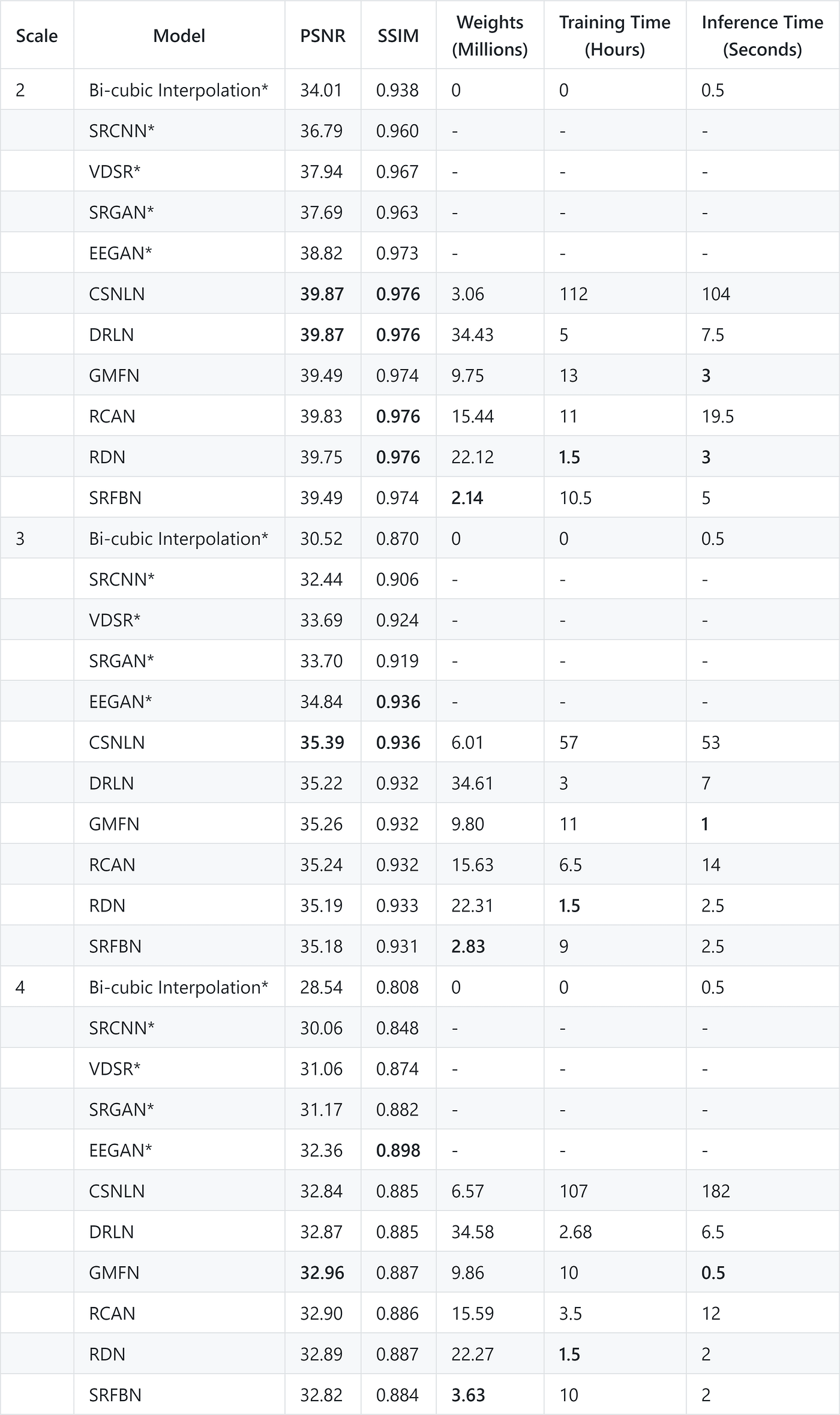Table 1. Comparison of the CNN-Based Single-Image Super-Resolution techniques, regarding reconstruction quality, no. of learnable parameters, training time, and inference time. The best value for each metric has been bolded. VDSR, SRGAN, and EEGAN are not discussed in this article. Refer to , , and , respectively.

--

--